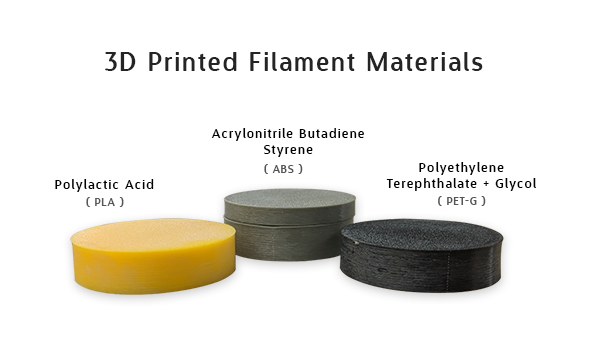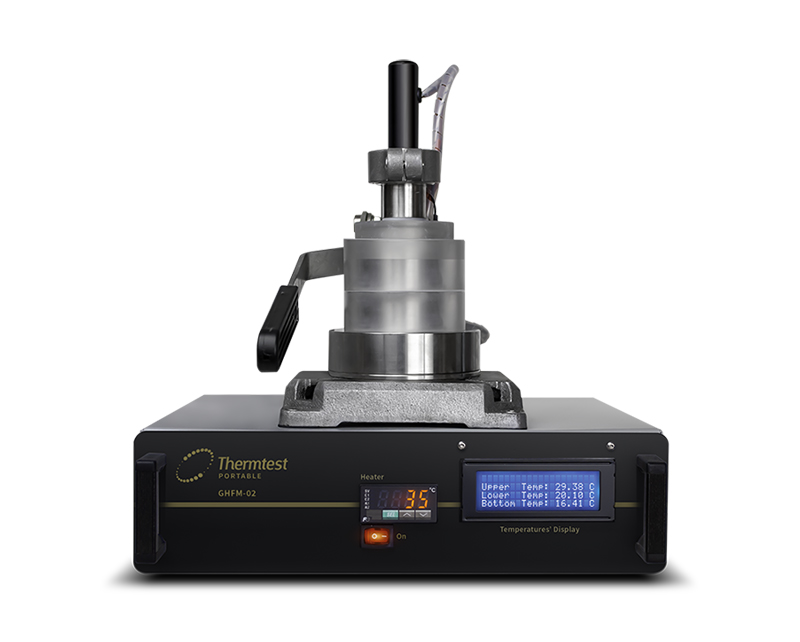The thermal conductivity (W/m•K) of 3D printed materials such as PLA (Polylactic Acid), PET-G (Polyethylene Terephthalate + Glycol), and ABS (Acrylonitrile Butadiene Styrene) were measured at ambient temperature using the Guarded Heat Flow Meter method.Figure 1. PLA (Polylactic Acid), ABS (Acrylonitrile Butadiene Styrene), and PET-G (Polyethylene Terephthalate + Glycol)

In this technique, the sample is subjected to a steady-state temperature gradient in the axial direction and the thermal conductivity of the sample is obtained by measuring the temperature difference across it and one additional temperature. The GHFM-02 is used for measuring the thermal resistance and thermal conductivity of a wide variety of solids, such as metals, polymers, and composites. Figure 1. shows a GHFM-02, with a guard to limit the lateral heat loss.Figure 2. GHFM-02 a portable guarded heat flow meter

Before the measurement, calibration is required using reference samples of known thermal conductivity (and thickness), supplied with the instrument. From the known thermal conductivity (λ) and the thickness (d), the thermal resistance (Rs) is calculated as Rs = (d/λ). Once the thermal equilibrium of the reference sample subjected to a temperature gradient in the GHFM-02 is obtained, the three temperatures, TU (upper temperature), TL (lower temperature), and TH (temperature above heat sink) is recorded.

From these temperatures, the temperature differences, ΔTs = TU-TL and ΔTB = (TL-TH) is calculated and the ratio of ΔTs/ΔTB is found.

A graph of Rs as a function of ΔTs/ΔTB is plotted using the reference sample to obtain the calibration curve. Using this calibration curve, the Rs value of the unknown sample can be obtained from the measured temperature differences, as mentioned above. The thermal conductivity of the unknown sample can then be calculated using the equation Rs = (d/λ).

## Measurement Results

The table shows the thermal conductivity of the 3D printed materials measured using the GHFM-02. The ambient condition during the measurement was 27°C .

Material Thickness (mm) Thermal Conductivity (W/m•K)
PLA 6.57 0.18
12.75 0.21
PET-G 6.47 0.18
12.61 0.22
ABS 6.43 0.17
12.54 0.19